### Home > CALC > Chapter 11 > Lesson 11.2.3 > Problem11-70

11-70.
1. Consider the infinite series below. For each, decide if it converges or diverges and justify your conclusion. State the test you used. Homework Help ✎

1.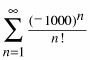2.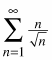3.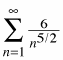4.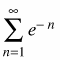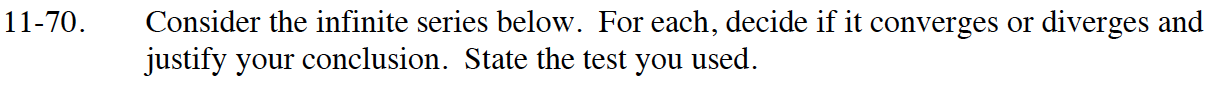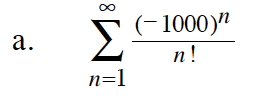Use the Ratio Test.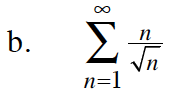$\frac{n}{\sqrt{n}}=\sqrt{n}$

$\lim_{n\to\infty}\sqrt{n}=?$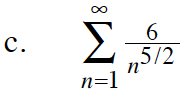This is a p-series.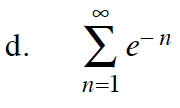This is a geometric series.